# PSEB 9th Class Science Solutions Chapter 8 Motion

Punjab State Board PSEB 9th Class Science Book Solutions Chapter 8 Motion Textbook Exercise Questions and Answers.

## PSEB Solutions for Class 9 Science Chapter 8 Motion

PSEB 9th Class Science Guide Motion Textbook Questions and Answers

Question 1.
An athelete completes one round of circular track of diameter 200 m in 40 s. What will be the distance covered and the displacement at the end of 2 min 20 s?
Solution: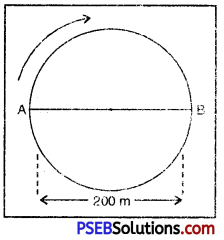Diameter of circular track (d) = 200m
Radius of circular track (r) = $$\frac{d}{2}$$ = $$\frac{200m}{2}$$ = 100m
Length of circular track (circumference) = 2πr = 2 × $$\frac{22}{7}$$ × 100
= $$\frac{4400}{7}$$m
Time taken to complete 1 round (t) = 40 s
Total time = 2 minutes 20 seconds
= (2 × 60 + 20) seconds
= (120 + 20) seconds
= 140 s.
Distance covered in 40 s = $$\frac{4400}{7}$$ m = (Circumference of 1 complete circular track)
Distance covered in 1 s = $$\frac{4400}{7×40}$$ m
Distance covered in 140 s = $$\frac{4400}{7×40}$$ × 140 = 2200 m
An athelete starting from A and going in clockwise direction returns to point A in 3 rounds and reaches point B in 3.5 rounds.
∴ Displacement in 3.5 rounds = AB = shortest distance between initial and final position = 200 m from A to B.Question 2.
Joseph jogs from end A to the other end B of a straight 300 m road in 2 minutes 50 seconds and then turns around and jogs 100 m back to point C in another 1 minute. What are Joseph’s average speeds and velocities in jogging (a) from A to B (b) from A to C?
Solution:
(a) Length between end point A and end point B (AB) = 300 m
Time taken (t) = 2 min. 30 s
= (2 × 60 + 30) s
= (120 + 30) s
= 150 s.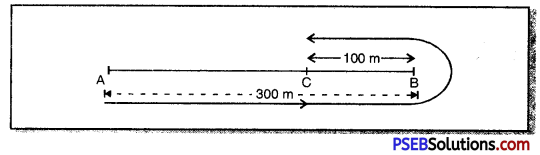Average speed = Average velocity
= $$\frac{Total distance between A and B(AB)}{Total time(t)}$$
= $$\frac{300m}{150s}$$
= 2ms-1

(b) Length from end A to end B + Length on return from B to point C.
= AB + BC
= 300 m + 100 m
= 400 m
Total Time = 2 min 30 s + 1 min
= 3 min 30 s
= (3 × 60 + 30) s
= (180 + 30) s
= 210 s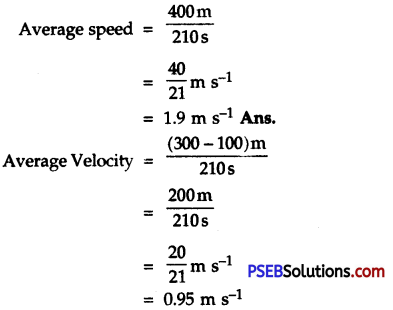Question 3.
Abdul while driving to school, computes the average speed for his trip to be 20 km h-1. On his trip along the same route, there is less traffic and average speed is 40 km h-1. What is the average speed for Abdul’s trip?
Solution: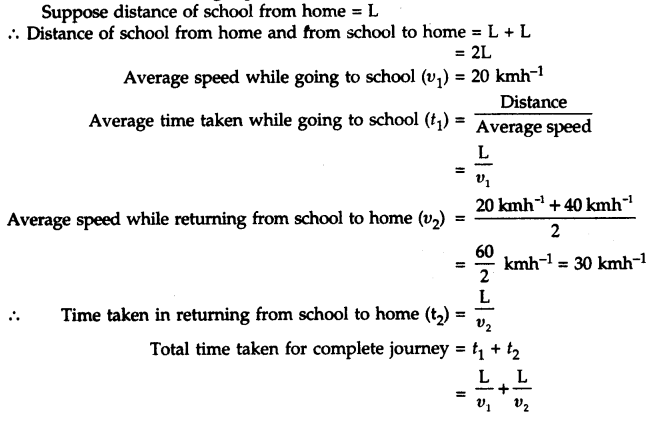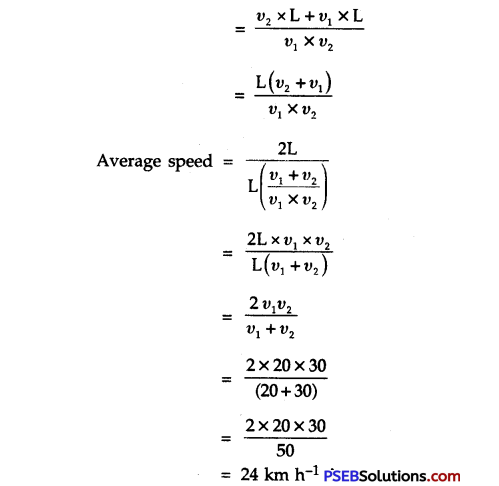Question 4.
A motorboat starting from rest on a lake accelerates in a straight line at a constant rate of 3.0 m s-2 for 8.0 s. How far does the boat travel during this time?
Solution:
Here, initial velocity of motorboat (u) = 0 [Starting from rest]
Acceleration (a) = 3.0 m s-2
Time (t) = 8.0 s
Distance covered by the motorboat (S) =?
We know, S = ut + $$\frac{1}{2}$$at2
= 0 × 8 + $$\frac{1}{2}$$ × 3 × (8)2
= 0 + $$\frac{1}{2}$$ × 3 × 8 × 8
∴ S = 96 m.
In other words, the motorboat covers a distance (S) = 96 m.

Question 5.
A driver of a car travelling at 52 kmh-1 applies the brakes and accelerates uniformly in opposite direction. The car stops in 5 s. Another driver going at 3 km h-1 applies his brakes slowly and stops in 10 s. On the same graph paper plot the speed versus time graph for the two cars. Which of the two cars travelled farther after the brakes were applied?
Solution:
In the figure AB and CD represent velocity-time graphs of two cars which have their speeds 52 kmh-1 and 30 kmh-1 respectively.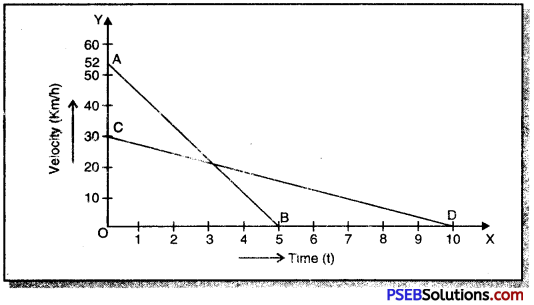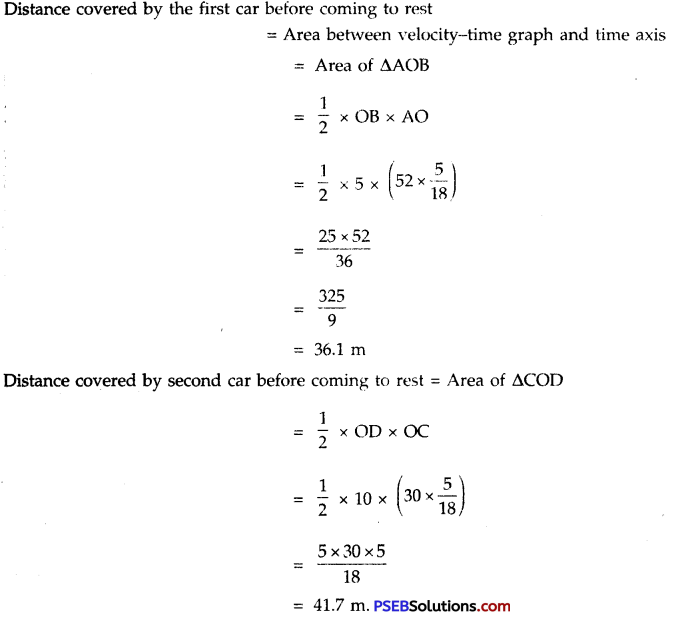In this way, after applying brakes the second car would cover more distance than the first car.

Question 6.
Fig shows the distance-time graphs of three objects A, B and C. Study the graph and answer the following questions: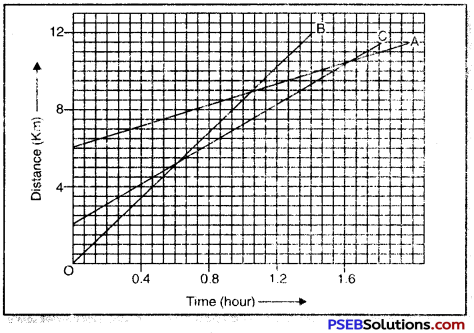(a) Which of the three is travelling the fastest?
(b) Are all three ever at the same point on the road?
(c) How far has C travelled when B passes A?
(d) How far has B travelled by the time it passes C?
Solution:
(a) Velocity of A = Slope of PN
$$\frac{10-6}{1.1-0}$$
$$\frac{40}{11}$$ = 3.63 kmh-1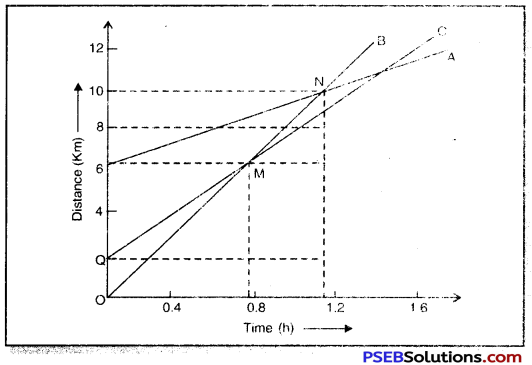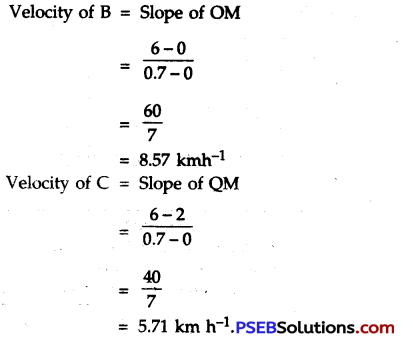Because slope of object B is maximum of all therefore, it is moving fastest.
(b) Since all the three graphs do not intersect at any point therefore, all the three do not meet ever at the same point on the road.
(c) When the object B passes A at point E (at 1.4 hr) then at that time the object C will be at F i.e. 9.3 km away from the origin O.
(d) B passes C at G after covering 8 km.Question 7.
A ball is gently dropped from a height of 20 m. If its velocity increases uniformly at the rate of 10 m s-2, with what velocity it will strike the ground?After what time will it strike the ground?
Solution:
u = 0 ms-1
S = 20 m
a = 10 ms-2
υ = ?
t = ?

Using υ2 – u2 = 2as
υ2 – (0)2 = 2 × 10 × 20
υ2 = 4000
∴ υ = $$\sqrt{400}$$
= $$\sqrt{20 \times 20}$$
= 20 m s-1
Now υ = u + at
20 = 0 + 10 × t
or t = $$\frac{20}{10}$$
∴ t = 2 s

Question 8.
Speed-time graph for a car is shown in the fig.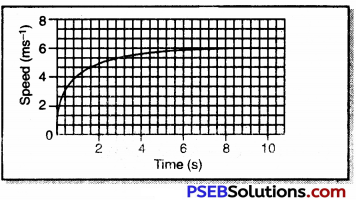(a) Find how far the car travelled in first 4 s. Shade the area on the graph that represents the distance travelled by car during this period.
(b) Which part of the graph represents uniform motion of the car?
Solution: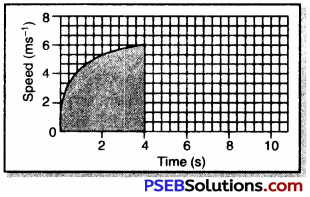(a) 5 small squares of x axis = 2s
3 small squares of y axis = 2 ms-1
Area of 15 small squares = 2s × 2 ms-1 = 4m
∴ Area of 1 small square = $$\frac{4}{15}$$
Area of velocity-time graph under 0 to 5s = 57 complete small squares + $$\frac{1}{2}$$ × 6 small squares.
= (57 + 3) small squares
= 60 small squares.
Distance covered by car in 4 s = 60 × $$\frac{4}{15}$$ m
= 16 m

(b) After 6 s the car has uniform motion.

Question 9.
State which of the following situations are possible and give an example for each of these.
(a) an object with a constant acceleration but with zero velocity.
(b) an object moving in a certain direction with an acceleration in the perpendicular direction.
(a) Yes, this situation is possible.
Example: When an object is projected upwards, its velocity at the maximum height is zero although acceleration on it is 9.8 ms-2 i.e. equal to g.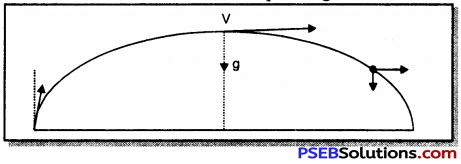(b) Yes, at the maximum height of projection the velocity is in the horizontal direction and its acceleration is perpendicular to the direction of motion as shown in figure.

Question 10.
An artificial satellite is moving in a circular path orbit of radius 42,250 km. Calculate its speed if it takes 24 hours to revolve around the earth.
Solution:
Radius of circular path of artificial satellite (r) = 42,250 km
Angle formed (subtended) at the centre of earth (θ) = 2π radian
Time taken by the satellite to complete 1 revolution (t) = 24hrs
= 24 × 3600s
= 86400 s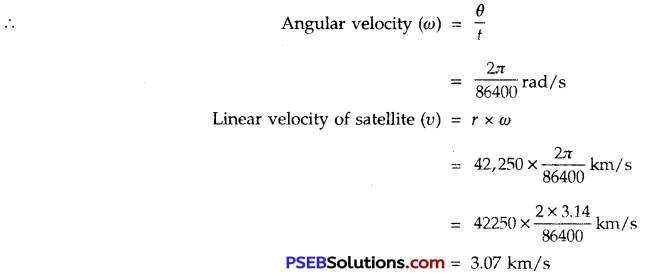Science Guide for Class 9 PSEB Motion InText Questions and Answers

Question 1.
An object has moved through a distance. Can it have zero displacements? If yes,support your answer with an example.
Yes, a body can have zero displacement, if fhis body While moving occupies its final position coinciding with its initial position.
Example: Suppose a body starting its motion from initial position O covers some distance and reaches a position A. If this body while moving returns to its initial position O then in that situation its displacement will be zero.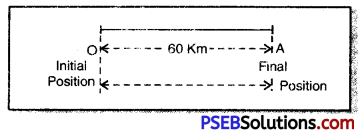But distance covered by the body = OA + AO
= 60 km + 60 km
= 120 kmQuestion 2.
A farmer moves along the boundary of a square field of side 10 m in 40 s. What will be the magnitude of displacement of the farmer at the end of 2 minutes 20 seconds?
Solution:
Total distance round the boundary of field once (i.e. circumference)
= AB + BC + CD + DA
= 10 m + 10 m + 10 m + 10 m = 40 m
Time taken to go round the field once = 40 s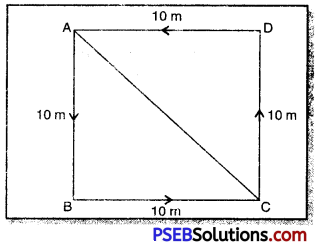Total time taken = 2minutes 20 seconds
= (2 × 60 + 20) seconds
= (120 + 20) seconds
= 140 seconds.
Time taken by fanner to complete 3 rounds of field = 3 × 40 s = 120 s
Time left after completing 3 rounds of field = (140 – 120)s = 20 s
∴ Distance covered by farmer in 40 s = 40 m
∴ Distance covered in 1 s = 1 m
Distance that would be covered in 20 s = 20 m
In other words farmer starting from point A and while going along the boundary of the field and after completing 3 rounds in 2 min 20 s would reach the point C.
∴ Displacement = AC
(the shortest distance between initial and final position)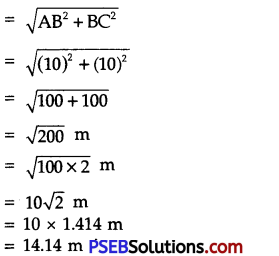Question 3.
Which of the following is true for displacement?
(a) It cannot be zero
(b) Its magnitude is greater than the distance travelled by the object.
(c) Its magnitude is less than or equal to distance travelled by the object.
(c) Its magnitude is less than or equal to distance travelled by the object.Question 4.
Distinguish between speed and velocity.
Distinction between Speed and Velocity:

 Speed Velocity 1. It is defined as the rate of a change of a position of a body i.e. the distance covered by a body per unit time. It is defined as the rate of change of displacement of a body. i.e. it is the speed in a particular direction. 2. It is a scalar quantity and can be completely represented by its magnitude only. It is a vector quantity. To represent it completely it requires both magnitude and direction. 3. Speed of an object is always positive. Velocity of an object can be both positive and negative.

Question 5.
Under what condition(s) is the magnitude of average velocity of an object is equal to its average speed?
We know, Average speed = Total distance travelled / Total time taken
and Average velocity = Displacement /Total time
When a body travels in a straight line with variable motion in the same direction then total distance covered and displacement are equal in magnitude. In this case the average speed and average velocity are equal.

Question 6.
What does the odometer of an automobile measure?
The odometer of an automobile measures the distance covered by it.

Question 7.
What does the path of an object look like when it is in uniform motion?
When an object is in uniform motion, it moves along a straight line. But an object can also move with uniform motion along a circular path.

Question 8.
During an experiment, a signal from a spaceship reached the ground station in five minutes. What was the distance of the spaceship from the ground station?The signal travels at a speed of light that is 3 × 10s ms-1.
Solution:
Time taken by the signal to reach the ground station from spaceship (t) = 5 min = 5 × 60 s = 300 s
Speed of Signal (υ) = Speed of light = 3 × 108 ms-1
Distance of the spaceship from earth (s) = ?
Distance of spaceship from ground (s) = speed of signal (υ) × Time (t)
= 3 × 108 × 300
= 3 × 108 × 3 × 102
= 9 × 108 × 102
= 9 × 1010 mQuestion 9.
When will you say a body is in:
1. uniform acceleration?
2. non-uniform acceleration?
1. Uniform Acceleration. When a body travels in a straight line and its velocity changes by equal amounts in equal intervals of time then it is said to travel with uniform acceleration.
2. Non-Uniform Acceleration. When the velocity of a body changes by unequal amounts in equal intervals of time then the body is said to travel with non-uniform acceleration.

Question 10.
A bus decreases its speed from 80 km h-1 to 60 km h-1 in 5 s. Find the acceleration of the bus.
Solution: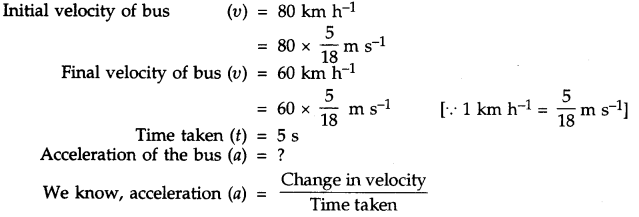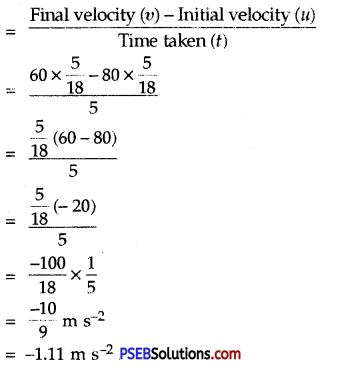Hence, the bus has negative acceleration (retardation).

Question 11.
A train starting from a railway station and moving with uniform acceleration attains a speed 40 km h-1 in 10 minutes. Find its acceleration.
Solution: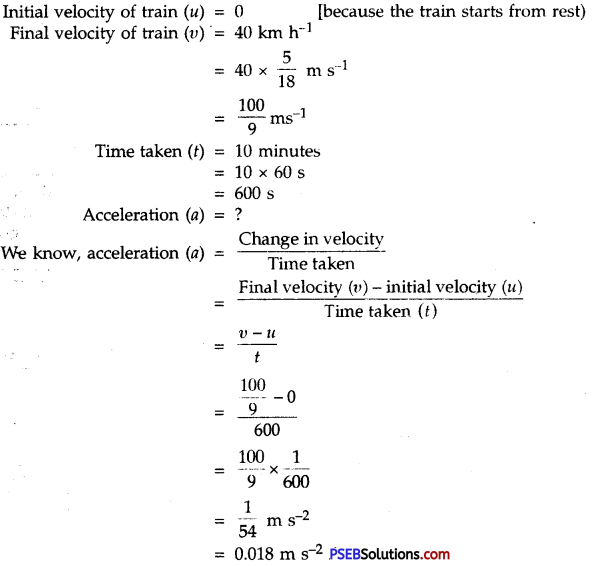Question 12.
What is the nature of the distance-time graphs (x – t) for uniform and non-uniform motion of an object?
When a body covers equal distances in equal intervals of time, then it is said to travel with uniform motion. In this situation, the distance covered by the body is directly proportional to the time taken. Therefore, distance-time (x – t) graph for uniform motion is a straight line.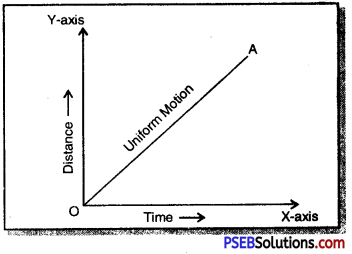Distance – time (x – t) graph for non-uniform motion may be a curved graph of any shape because a body travels unequal distances in equal intervals of time.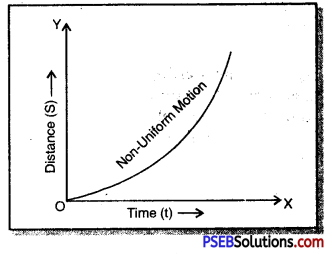Question 13.
What can you say about the motion of object whose distance – time graph is a straight line parallel to time axis?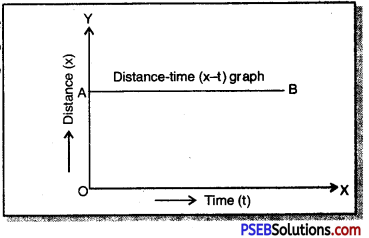The object whose distance-time (x – t) graph is a straight line parallel to the time axis will be at rest with respect to the surroundings.

Question 14.
What can you say about the motion of an object if its speed-time graph is a straight line parallel to time axis?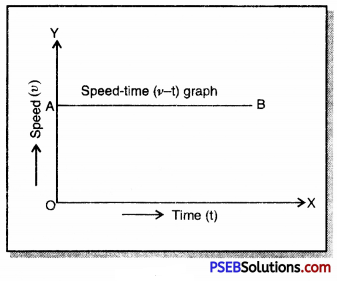The object whose speed – time (u – t) graph is a straight line parallel to time axis shows that it is in motion with uniform speed.Question 15.
What is the quantity which is measured by the area occupied below velocity-time graph?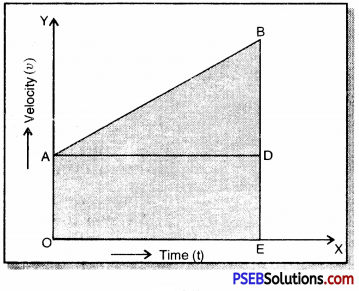The area occupied below velocity-time graph measures displacement of the body.

Question 16.
A bus starting from rest moves with a uniform acceleration of 0.1 ms-2 for two minutes. Find (a) the speed acquired (b) the distance travelled.
Solution:
(a) Initial speed of the bus (u) = 0 (Starting from Rest)
Acceleration of the bus (a) = 0.1 m s-2
Time taken (t) = 2 minutes
= 2 × 60 s
= 120 s
Final speed of the bus (υ) = ?
Distance travelled by the bus (S) =?
We know, υ = u + at
υ = 0 + 0.1 × 120
υ = 1 × 12
υ = 12 ms-1

(b) Again, using S = ut + $$\frac{1}{2}$$ at2
S = 0 × 120 + $$\frac{1}{2}$$ × 0.1 × (120)2
= 0 + $$\frac{1}{2}$$ × 0.1 × 120 × 120
= $$\frac{1}{2}$$ × 1 × 12 × 120
= 720 m/s

Question 17.
A train is travelling at a speed of 90 km h-1. Brakes are applied so as to produce a uniform acceleration of -0.5 ms-2. Find how far the train will move before it is brought to rest?
Solution:
Initial speed of train (υ) = 90km h-1
= 90 × $$\frac{5}{18}$$ m s-1
= 5 × 5 ms-1
= 25 ms-1
Uniform acceleration (a) = – 0.5m s-2
Final speed of the train (υ) = 0
Distance moved by the train (S) =?
We know, υ2 – u2 = 2as
(0)2 – (25)2 = 2 × (-0.5) × S
– 25 × 25 = -1 × S
∴ S = 625 mQuestion 18.
A trolley, while going down an inclined plane has an acceleration of 2 cm s~2. What will be its velocity 3 s after the start?
Solution:
Here initial velocity of trolley (u) = 0 [∵ starting from rest]
Acceleration (a) = 2cm s-2
Time (t) = 3 s
Final velocity of trolley (υ ) = ?
We know, υ = u + at
υ = 0 + 2 × 3
∴ Final velocity of trolley (υ) = 6 cm s-1 Ans.

Question 19.
A racing car has uniform acceleration of 4 ms-2. What distance will it cover in 10 s after start?
Solution:
Acceleration of racing car (a) = 4 ms-2
Initial velocity of racing car (u) = 0
Time (t) = 10 s
Distance covered by the car (S) = ?
We know, S = ut + $$\frac{1}{2}$$at2
S = 0 × 10 + $$\frac{1}{2}$$ × 4 × (10)2
S = 0 + 2 × 10 × 10
∴ Distance covered by racing car (S) = 200 m

Question 20.
A stone is thrown in a vertically upward direction with a velocity of 5 m s-1. If the acceleration of the stone during its motion is 10 m s-2 in the downw ard direction. What will be the height attained by the stone and how much time will it take to reach there?
Solution:
Here, initial velocity (u) = 5 m s-1
Acceleration (a) = – 10 ms-2
[∵ it moves upward against the gravity]
Final velocity of stone (υ) = 0 [At the highest point it is brought to rest]
Height attained (S = h) = ?
Time taken (t) =?
We know,
υ = u + at
0 = 5 + (-10) × t
0 = 5 – 10 × t
10 × t = 5
or t = $$\frac{5}{10}$$
∴ Time taken (t) = 0.5 s
Again, using υ2 – u2 = 2as
(0)2 – (5)2 = 2 × -10 × h
– 5 × 5 = – 20 × h
or h = $$\frac{-25}{-20}$$ = $$\frac{5}{4}$$
∴ Height attained (h) – 1.25 m